# 《R语言游戏数据分析与挖掘》一3.2　低级绘图函数

+关注继续查看

### 3.2　低级绘图函数

R还可以通过函数在现有图形的基础上增加一些额外的内容，如添加标题、绘制坐标轴、在特定的位置增加图形（如辅助线，拟合线）或文字等。这些函数在R中称为低级作图命令（low-level plotting command）。

### 3.2.1　标题

> attach(iris)
> boxplot(Sepal.Length~Species,col=heat.colors(3),
+         main=list("Sepal.Length按照Species分类的箱线图",
+                   font=4,col="red",cex=1.5),
+         sub=list("数据来源：iris数据集",font=3,
+                  col="green",cex=0.8),
+         xlab="Species",ylab="Sepal.Length")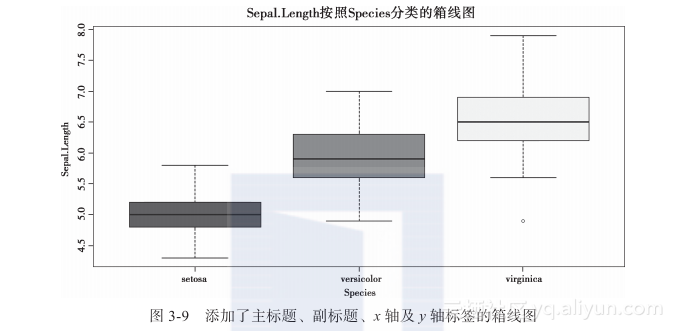title(main = NULL, sub = NULL, xlab = NULL, ylab = NULL,
line = NA, outer = FALSE, ...)


title常用参数说明如表3-4所示。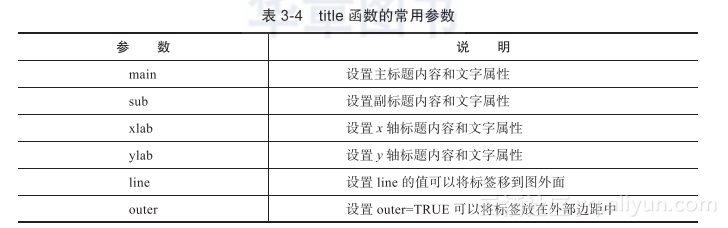> boxplot(Sepal.Length~Species,col=heat.colors(3))
> title(main=list("Sepal.Length按照Species分类的箱线图",
+                      font=4,col="red",cex=1.5),
+       sub=list("数据来源：iris数据集",font=3,
+                col="green",cex=0.8),
+       xlab="Species",ylab="Sepal.Length")


> boxplot(Sepal.Length~Species,col=heat.colors(3))
> title(main="Sepal.Length按照Species分类的箱线图",
+       font.main=4,col.main="red",cex.main=1.5,
+       sub="数据来源：iris数据集",font.sub=3,
+       col.sub="green",cex.sub=0.8,
+       xlab="Species",ylab="Sepal.Length")


#### 3.2.2　坐标轴

axis(side, at = NULL, labels = TRUE, tick = TRUE, line = NA,
pos = NA, outer = FALSE, font = NA, lty = "solid",
lwd = 1, lwd.ticks = lwd, col = NULL, col.ticks = NULL,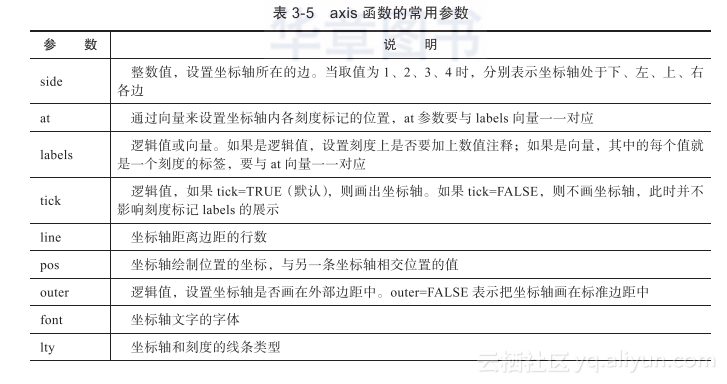> #加载iris数据到内存
> attach(iris)
> #绘制箱线图
> boxplot(Sepal.Length~Species,col=heat.colors(3),
+           axes=FALSE,xlab="Species",ylab="Sepal.Length")
> #设置X轴样式
> axis(side=1,at=1:3,labels=unique(Species),col.axis="red",tick=FALSE)
> #设置Y轴样式
> axis(side=2,col.ticks = "gold",font = 3,col = "blue")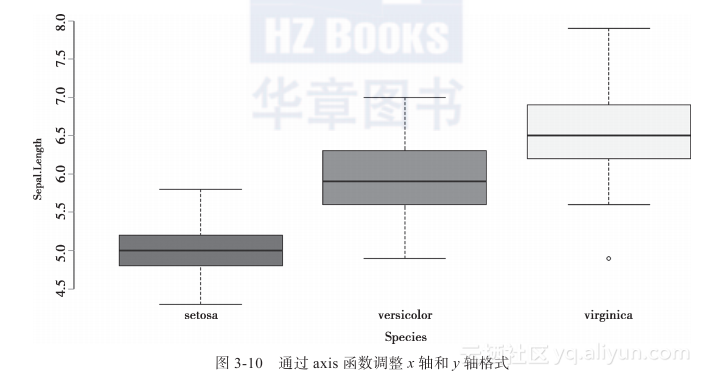side=1表示设置x轴，col.axis="red"b表示将x轴刻度标记颜色设置为红色，tick=FALSE表示不显示坐标轴；side=2表示设置y轴，font = 3表示y轴字体是斜体，col = "blue"表示y轴线条是蓝色，col.ticks = "gold"表示将刻度颜色设置为金色。

#### 3.2.3　图例

legend函数的常用参数如表3-6所示。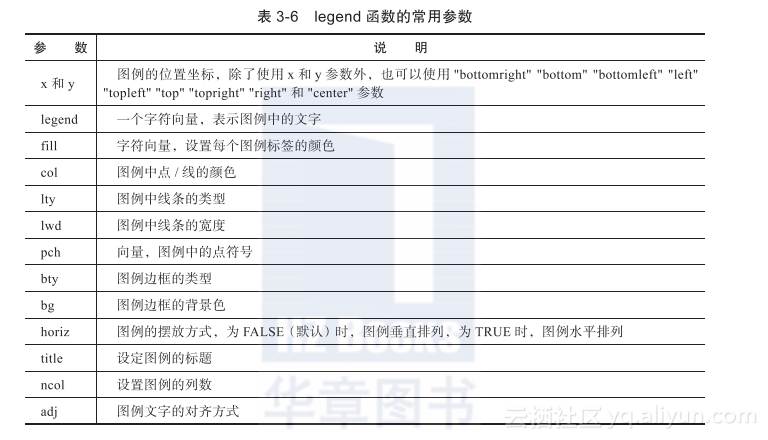> #绘制分组柱状图
> # 添加图例
+         ncol=5,fill=cm.colors(5),bty="n")


#### 3.2.4　网格线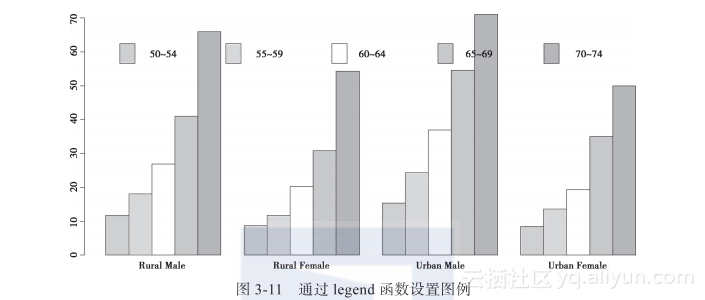> op <- par(mfcol=1:2)
> grid()
> grid(NA,7,lty=2,lwd=1.5,col="green")
> par(op)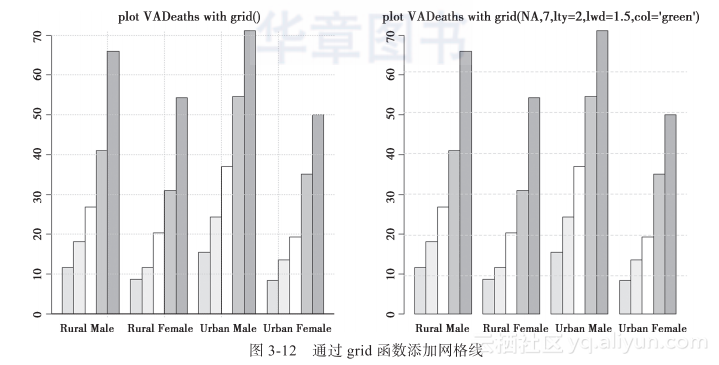3.2.5　点

points(x, y = NULL, type = "p", ...)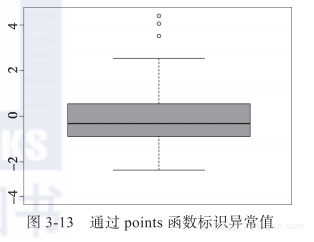> set.seed(1234)
> data <- c(rnorm(100,mean=0,sd=1),rnorm(3,mean=4,sd=1))    #创建数据对象data
> boxplot(data,col="violet",ylim=c(-4,5),outline=F)         #绘制箱线图
> points(rep(1,3),data[101:103],pch=21,bg="yellow",cex=1.2) #标识异常值


#### 3.2.6　文字

text(x, y = NULL, labels = seq_along(x), adj = NULL,
pos = NULL, offset = 0.5, vfont = NULL,
cex = 1, col = NULL, font = NULL, ...)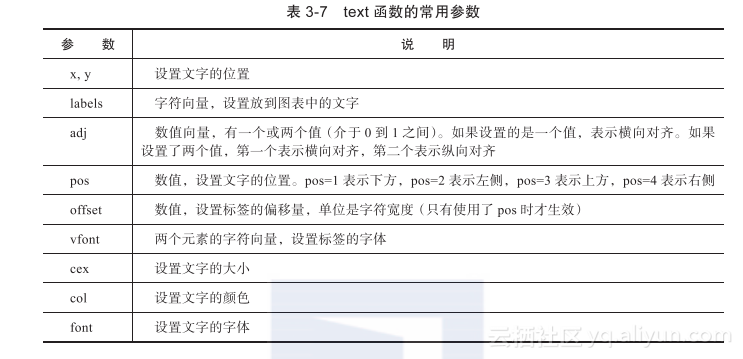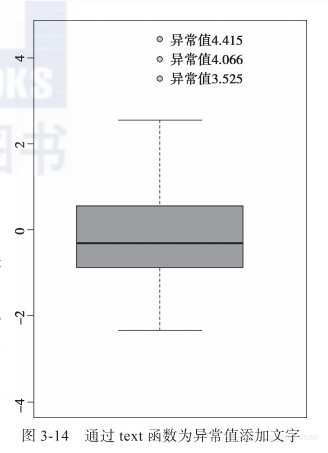> text(rep(1,3),data[101:103],pos=4,label=paste0("异常值",data[101:103]))#pos=4表示在右侧


#### 3.2.7　线

1. lines函数绘制曲线
lines函数可以在已有图形中添加曲线，使用格式为lines(x, y = NULL, type = "l", ...)。plot函数的很多参数也可用于lines。一些比较有用的参数包括lty（线条类型）、lwd（线条宽度）、col（线条颜色）等。

> data(economics, package = "ggplot2")
> attach(economics)                #将economics加载到内存
> plot(date,psavert,type="l",ylab="",ylim=c(0,26))    #绘制psavert随时间变化的时序图
> lines(date,uempmed,type="l",col="blue")    #绘制uempmed曲线，并设置为蓝色
> detach(economics)                #将economics从内存中移除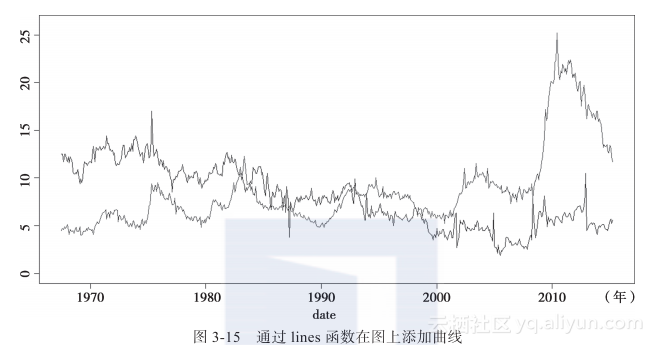1. abline函数绘制直线
使用abline函数可以在已有图形中添加参考直线。abline函数的基本形式是：
abline(a = NULL, b = NULL, h = NULL, v = NULL, reg = NULL,
coef = NULL, untf = FALSE, ...)


abline函数的参数如表3-8所示。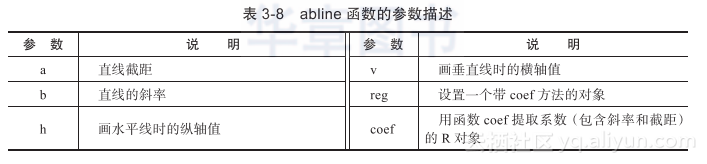> attach(iris)
> # 绘制一幅简单的散点图
> plot(Petal.Length~Petal.Width)
> # 绘制Petal.Length变量均值的水平线
> abline(h=mean(Petal.Length),col="gray60")
> # 绘制Petal.Width变量均值的竖直线
> abline(v=mean(Petal.Width),col="gray60")
> # 绘制拟合直线
> abline(lm(Petal.Length~Petal.Width),
+          col="red",lwd=2,lty=3)
> detach(iris)|
JavaScript 前端开发 数据挖掘
《R语言游戏数据分析与挖掘》一2.1　开发环境准备和快速入门

1869 0
|

《R语言游戏数据分析与挖掘》一导读

1794 0
|

《R语言游戏数据分析与挖掘》一3.4　小结

1083 0
|

《R语言游戏数据分析与挖掘》一3.3　高级绘图函数

3094 0
|
JavaScript 前端开发 数据挖掘
《R语言游戏数据分析与挖掘》一3.1　常用图形参数

1498 0
|

《R语言游戏数据分析与挖掘》一第3章 R语言绘图重要技术

1048 0
|

《R语言游戏数据分析与挖掘》一2.4　小结

1155 0
|

《R语言游戏数据分析与挖掘》一2.2　数据对象

1734 0
|

《R语言游戏数据分析与挖掘》一第2章 必备R语言基础

1052 0
|

《R语言游戏数据分析与挖掘》一1.4　小结

964 0